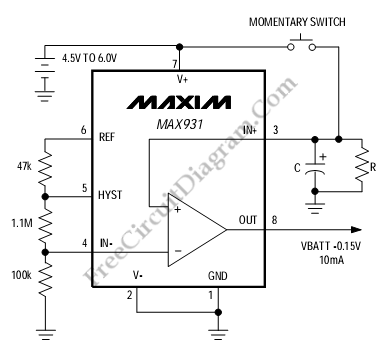# Auto-Off Power SourceThis is a Auto-Off Power Source circuit. This circuit is used to turn off the power source automatically. This circuit uses the MAX931 that has key features such as high current output, an internal reference, 2.5µA supply current, and hysteresis. This circuit requires only 3.5uA quiescent current to provide voltage of Vbatt -0.12V with 10mA load. Here is the circuit:If we use the component values given, the maximum hysteresis is ±50mV which programed by the three-resistor voltage divider and the IN-voltage is set at 100mV. So, for IN+ falling,the IN+trip threshold is approximately 50mV.

This circuit also uses RC time constant to determines the maximum power-on time of the OUT pin before power-down occur. The period is defined by following equation:
T=R x C x 4.6sec

[Source: MAXIM Application Note]